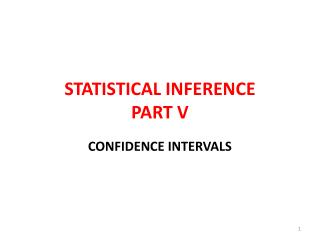DownloadDownload PresentationSTATISTICAL INFERENCE PART V

# STATISTICAL INFERENCE PART V

Download Presentation## STATISTICAL INFERENCE PART V

- - - - - - - - - - - - - - - - - - - - - - - - - - - E N D - - - - - - - - - - - - - - - - - - - - - - - - - - -
##### Presentation Transcript

1. STATISTICAL INFERENCEPART V CONFIDENCE INTERVALS

2. INTERVAL ESTIMATION • Point estimation of  : The inference is a guess of a single value as the value of . No accuracy associated with it. • Interval estimation for  : Specify an interval in which the unknown parameter, , is likely to lie. It contains measure of accuracy through variance.

3. INTERVAL ESTIMATION • An interval with random end points is called a random interval. E.g., is a random interval that contains the true value of  with probability 0.95.

4. Interpretation ( ) ( ) ( ) ( ) ( ) ( ) ( ) ( ) ( ) ( ) μ (unknown, but true value) 90% CI  Expect 9 out of 10 intervals to cover the true μ

5. INTERVAL ESTIMATION • An interval (l(x1,x2,…,xn), u(x1,x2,…,xn)) is called a 100 % confidence interval (CI) for  if where 0<<1. • The observed values l(x1,x2,…,xn) is a lower confidence limit and u(x1,x2,…,xn) is an upper confidence limit. The probability is called the confidence coefficient or the confidence level.

6. INTERVAL ESTIMATION • If Pr(l(x1,x2,…,xn))= , then l(x1,x2,…,xn) is called a one-sided lower100 % confidence limit for  . • If Pr( u(x1,x2,…,xn))= , then u(x1,x2,…,xn) is called a one-sided upper100 % confidence limit for  .

7. METHODS OF FINDING PIVOTAL QUANTITIES • PIVOTAL QUANTITY METHOD: If Q=q(x1,x2,…,xn) is a r.v. that is a function of only X1,…,Xnand, and if its distribution does not depend on  or any other unknown parameter (nuisance parameters), then Q is called a pivotal quantity. nuisance parameters: parameters that are not of direct interest

8. PIVOTAL QUANTITY METHOD Theorem: Let X1,X2,…,Xn be a r.s. from a distribution with pdf f(x;) for  and assume that an MLE (or ss) of  exists: • If  is a location parameter, then Q=  is a pivotal quantity. • If  is a scale parameter, then Q= / is a pivotal quantity. • If 1 and 2 are location and scale parameters respectively, then are PQs for 1 and  2.

9. Note • Example: If 1 and 2 are location and scale parameters respectively, then is NOT a pivotal quantity for 1 because it is a function of 2 A pivotal quantity for 1 should be a function of only 1 andX’s, and its distribution should be free of 1 and 2 .

10. Example • X1,…,Xn be a r.s. from Exp(θ). Then, is SS for θ, and θ is a scale parameter. • S/θ is a pivotal quantity. • So is 2S/θ, and using this might be more convenient since this has a distribution of χ²(2n) which has tabulated percentiles.

11. CONSTRUCTION OF CI USING PIVOTAL QUANTITIES • If Q is a PQ for a parameter  and if percentiles of Q say q1 and q2 are available such that Pr{q1 Q q2}=, Then for an observed sample x1,x2,…,xn; a 100% confidence region for  is the set of  that satisfy q1 q(x1,x2,…,xn;)q2.

12. EXAMPLE • Let X1,X2,…,Xn be a r.s. of Exp(),>0. Find a 100 % CI for  . Interpret the result.

13. EXAMPLE • Let X1,X2,…,Xn be a r.s. of N(,2). Find a 100 % CI for  and 2 . Interpret the results.

14. APPROXIMATE CI USING CLT • Let X1,X2,…,Xn be a r.s. • By CLT, Non-normal random sample The approximate 100(1−)% random interval for μ: The approximate 100(1 −)% CI for μ:

15. APPROXIMATE CI USING CLT • Usually,  is unknown. So, the approximate 100(1)% CI for : Non-normal random sample • When the sample size n ≥ 30, t/2,n-1~N(0,1).

16. Confidence level Graphical Demonstration of the Confidence Interval for m 1 - a Upper confidence limit Lower confidence limit

17. Standard Normal Student t 0 Inference About the Population Mean when  is Unknown • The Student t Distribution

18. Student t with 30 DF Student t with 2 DF Student t with 10 DF 0 Effect of the Degrees of Freedom on the t Density Function The “degrees of freedom” (a function of the sample size) determines how spread the distribution is compared to the normal distribution.

19. Degrees of Freedom t.100 t.05 t.025 t.01 t.005 3.078 1.886 1.638 1.533 1.476 1.440 1.415 1.397 1.383 1.372 1.363 1.356 6.314 2.920 2.353 2.132 2.015 1.943 1.895 1.860 1.833 1.812 1.796 1.782 12.706 4.303 3.182 2.776 2.571 2.447 2.365 2.306 2.262 2.228 2.201 2.179 31.821 6.965 4.541 3.747 3.365 3.143 2.998 2.896 2.821 2.764 2.718 2.681 63.657 9.925 5.841 4.604 4.032 3.707 3.499 3.355 3.250 3.169 3.106 3.055 1 2 3 4 5 6 7 8 9 10 11 12 0 t0.05, 10 = 1.812 Finding t-scores Under a t-Distribution (t-tables) .05 t 1.812

20. EXAMPLE • A new breakfast cereal is test-marked for 1 month at stores of a large supermarket chain. The result for a sample of 16 stores indicate average sales of \$1200 with a sample standard deviation of \$180. Set up 99% confidence interval estimate of the true average sales of this new breakfast cereal. Assume normality.

21. ANSWER • 99% CI for : (1067.3985, 1332.6015) With 99% confidence, the limits 1067.3985 and 1332.6015 cover the true average sales of the new breakfast cereal.

22. Checking the required conditions • We need to check that the population is normally distributed, or at least not extremely nonnormal. • Look at the sample histograms, Q-Q plots … • There are statistical methods to test for normality